Science Journal of Applied Mathematics and Statistics
Volume 4, Issue 6, December 2016, Pages: 249-255

Estimating the Extreme Financial Risk of the Kenyan Shilling Versus Us Dollar Exchange Rates

Charles Kithenge Chege1, Joseph Kyalo Mungat’u2, Oscar Ngesa3

1Applied Statistics, Department of Statistics and Actuarial Science, Jomo Kenyatta University of Agriculture and Technology, Nairobi, Kenya

2Statistics, Department of Statistics and Actuarial Science, Jomo Kenyatta University of Agriculture and Technology, Nairobi, Kenya

3Applied Statistics, Department of Statistics and Actuarial Science, Jomo Kenyatta University of Agriculture and Technology, Nairobi, Kenya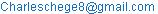(C. K. Chege)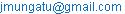(J. K. Mung’atu)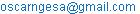(O. Ngesa)

Charles Kithenge Chege, Joseph Kyalo Mungat’u, Oscar Ngesa. Estimating the Extreme Financial Risk of the Kenyan Shilling Versus US dollar Exchange Rates. Science Journal of Applied Mathematics and Statistics. Vol. 4, No. 6, 2016, pp. 249-255. doi: 10.11648/j.sjams.20160406.11

Received: September 1, 2016; Accepted: September 23, 2016; Published: October 14, 2016

Abstract: In the last decade, world financial markets, including the Kenyan market have been characterized by significant instabilities. This has resulted to criticism on available risk management systems and motivated research on better methods capable of identifying rare events that have resulted in heavy consequences. With the high volatility of the Kenyan Shilling/Us dollar exchange rates, it is important to come up with a more reliable method of evaluating the financial risk associated with such financial data. In the recent past, extensive research has been carried out to analyze extreme variations that financial markets are subject to, mostly because of currency crises, stock market crashes and large credit defaults. We considered the behavior of the tails of financial series. More specially was focus on the use of extreme value theory to assess tail-related risk; we thus aim at providing a modeling tool for modern risk management. Extreme Value Theory provides a theoretical foundation on which we can build statistical models describing extreme events. This will help in predictability of such future rare events.

Keywords: Extreme Value Theory (EVT), Generalized Pareto Distribution (GPD), Peaks-Over-Threshold (POT)

Contents

1. Introduction

1.1. Background of the Study

In the study and practice of financial risk management, the Value at Risk (VaR) metric is one of the most widely risk measure. The volatility of foreign exchange rates can expose the portfolio of a financial institution to enormous risk. VaR summarizes these risks into a single number. For a given portfolio of assets, the N-day X-percent VaR is the loss amount V that the portfolio is not expected to exceed in the next N days with X-percent certainty. Proper estimation of VaR is necessary in that it needs to accurately capture the level of risk exposure that the firm is exposed to, but if it overestimates the risk level, then the firm will unnecessarily set aside capital to cover the risk, when the capital could have been better elsewhere. Investors and risk managers have become more concerned with events occurring under extreme market conditions. This proposal argues that EVT is a useful supplementary risk measure because it provides more appropriate distributions to fit extreme events. Unlike VaR methods, no assumptions are made about the nature of the original distribution of all the observations. Some EVT techniques can be used to solve for very high quantiles, which is very useful for predicting crashes and extreme situations.

1.2. Statement of the Problem

The volatility of exchange rates across the globe has been a problem due to lack of predictability. Most methods that have been applied in calculation of Value at Risk use normality assumptions. However, interest is on the behavior of extreme events than on the normal observations. This study comes up with a better method of modeling extremal events observed in the Kenyan Shilling versus US dollar exchange rates.

1.3. Justification of the Study

With success in finding an EVT method of modeling the volatility of exchange rates, it will be possible to predict the behavior of the Kenyan Shilling/Us dollar. Thus, firms that engage in international business can be able to prepare for shocks that are common with exchange rates.

1.4. Objectives

1.4.1. General Objective

To estimate the extreme financial risk associated with the Kenyan Shilling versus US Dollar exchange rates.

1.4.2. Specific Objective

i.        To fit a heteroskedastic model to the financial time series data.

ii.       To t an appropriate extreme value theory model to the data.

iii.     Find the VaR using the model for different levels of confidence.

2. Literature Review

The dynamic nature of foreign exchange behaviour is an acceptable phenomena and all participants in stock markets including regulators, professionals and academics have consensus about it. But what causes foreign exchange prices volatility is a questions that remains unsettled in the finance field. Because of the high number of variables that are involved, this is not an easy task and up to now, there is no consensus about it. However, researchers in the quest of answers to the problem of stock prices volatility have investigated this from different perspectives. From as late twentieth century, particularly after introduction of ARCH model by Engel (1982), several hundred researches that mainly accomplished in the developed countries and to some extent in developing countries have been done by researchers using different methodologies. As said by Bollerslev (1986) and Poon and Granger (2000).

Engel (1982) published a paper that measured the time-varying volatility. His model, ARCH, is based in the idea that a natural way to update variance forecast is to average it with the most recent "squared" (this is, the squared deviation of the rate of return from its mean). While conventional time series and economic models operate under an assumption of constant variance, the ARCH process allows the conditional variance to change over time as a function of past errors leaving the unconditional variance constant. In the empirical application of the ARCH model, a relatively long lag in the conditional variance equation is often called for, and to avoid problems with negative variance parameters a fixed lag structure is typically imposed. Bollerslev (1986), to overcome the ARCH limitation introduced his model, GARCH, which generalized the ARCH model to allow for both a longer memory and a more flexible lag structure. As ARCH models, a relatively long lag in the conditional variance equation is often called for, and to avoid problems with negative variance parameters a fixed lag structure is typically imposed. In the ARCH process, the conditional variance is specified as a linear function of past variance only, whereas the GARCH process allows lagged conditional variances to enter in the model as well.

Engle and Robins (1987) introduced the ARCH-mean model by extending the ARCH model to allow conditional variance to be the determinant of thee mean, whereas in its standard form, ARCH model expresses the conditional variance as a linear function of past square innovations, in this new model they hypothesize that, changing conditional variance directly affects the expected return on a portfolio. Their result from applying this model to three different data sets is quite promising. Consequently, they conclude that risk premia are not time invariant; rather they vary systematically with agent's perception of the underlying uncertainty. Nelson (1991) extended the ARCH framework in order to better describe the behavior of return volatility. Nelson's study is important because of the fact that it extended the ARCH methodology in a new direction, breaking the rigidness of the GARCH specification. The most important contribution was to propose a model E-GARCH to test the hypothesis that variance of return was in influenced differently by positive and negative excess returns. His study found that not only was the statement true, but also that excess returns were negatively related to stock market variance.

Glosten and Runkel (1993), to modify the primary restriction of GARCH-M model based upon the truth that GARCH model enforced a systematic response of volatility to positive and negative shocks, introduced GJR's (TGARCH) models. They conclude that there is a positive but significant relation between the conditional mean and conditional volatility of excess return on stocks when the standard GARCH-M framework is used to model the stochastic volatility of stock returns. On the other hand, Campbell’s Instrumental Variable Model estimates a negative relation between conditional mean and conditional volatility. They empirically show that the standard GARCH-M model is mis-specified and alternatively specifications provide reconciliation between these two results. When the model is modified to allow positive and negative unanticipated returns to have different impacts on conditional variance, they find that a negative relation between the conditional mean and the conditional variance of the excess return on stock. Finally, they also find that positive and negative expected returns have vastly different effects on future conditional variance and the expected impact of a positive unexpected return is negative.

Engle and Ng (1993) measure the impact of bad and good news on volatility and report an asymmetry in stock market volatility towards good news as compared to bad news. More specifically volatility is assumed to be associated with the arrival of news. A sudden drop in price is associated with bad news while a sudden increase in price is said to be due to good news. Engle and Ng (1993) find that bad news create more volatility than good news of equal importance. This asymmetric characteristic of market volatility has come to be known as the "leverage effects". The studies of Black (1976), Christie (1982), FSS (1987), Schwert (1990) and Pagan and Schwert (1989) also explain this volatility asymmetry with leverage effect". However, their models do not capture this asymmetry. Engle and Ng (1993) provide new diagnostic test and models, which incorporate the asymmetry between the type of news and volatility, they advise researchers to use such enhanced models when studying volatility. In many fields of modern science, engineering and insurance, extreme value theory is well established, e.g P. Embrechts and Mikosch (1999), Reiss et al. (2007). Recently, more and more research has been undertaken to analyze the extreme variations that financial markets are subject to, mostly because of currency crisis, stock market crashes and large credit defaults. The tail behavior of financial series has, among others, been analyzed in Koedijk and de Vries (1990), and Diebold and Stroughair (1970). An interesting discussion about the potential of extreme value theory in risk management is given in Diebold and Stroughair (1970).

While conditional models are superior for short-term forecasts, their value vanishes with increasing time horizon. Christoffersen and Diebold (2000) argue that the recent history of data series has little to tell about the probability of events occurring in the future. This applies especially to the prediction of rare events like disasters, which are assumed to be stochastically independent. Therefore, Jodeu and de Vries (2000) recommend deriving predictions about extreme events from unconditional distributions. Extreme value theory provides statistical tools to estimate the tails of probability distributions. A much more comprehensive treatment can be found in Embrechts and Kluppelberg (1997). They prove that the sample maximum of a distribution exhibiting fat tails converges towards the Frechet distribution. In accordance with the arguments of Jodeu and de Vries (2000), the subsequent application of extreme value theory is based on unconditional distributions regardless of the conditional heteroscedasticity in price changes. Several studies have shown Extreme Value Theory to be one of the best methods for application in Value at Risk (VaR) estimation.

3. Research Methodology

In this section, we discuss GARCH models and Extreme Value Theory models. Lastly, we consider the Value at Risk (VaR) as a risk measure.

3.1. Models of Volatility

ARCH models are capable of modeling and capturing many of the stylized facts of volatility behavior, usually observed in financial time series including time varying volatility or volatility clustering as stated by Zivot and Wang (2007). The serial correlation in squared returns or conditional Heteroskedasticity (volatility clustering) can be modeled using a simple Autoregressive (AR) process for squared residuals. For example, let rt, denote the stationary time series such as financial returns, the rt, can be expressed as its mean plus a white noise if there is no significant autocorrelation in rt itself:

rt = µ + εt                                    (1)

Where µ is the mean of rt, and εt is IID with mean zero.

An ARCH (p) model is specified by the equation:

σt201ε2t-1+…+αpε2t-p                       (2)

The problem with applying the original ARCH model is the non-negativity constrain on the coefficient parameter of the (βjs) to ensure the positivity of the conditional variance. However, when a model requires many lags to model the process correctly, the non-negativity maybe violated. To avoid the long lag structure of the ARCH (q) model developed by Engel (1982), Bollerslev (1986), generalized ARCH model, the so called GARCH, by including the lagged values of the conditional variance. Thus, GARCH (p, q) specifies the conditional variance to be a linear combination of (q) lags of the squared residuals εt-j2 from the conditional return equation and (p) lags from the conditional varianceσ2t−j. Then, the GARCH (p, q) specification can be written as follows:

rt = σtεt                                         (3)

σ2t0+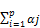εt-j2 +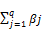σ2t-j                    (4)

3.2. The Mean Excess Graph

The mean excess function for the Generalized Pareto Distribution is linear (tends towards infinity). According to the results of Picklands, Balkema - in Haan, and for a high threshold, the excess over a threshold for a given series converges to a GPD. It is possible to choose the threshold where an approximation by the GPD is reasonable by detecting an area with shape on the graph. Another Graphical tool that will be used to choose the threshold is the Hill graph.

3.3. Threshold Determination

3.3.1. The Mean Excess Graph

The mean excess function for the Generalized Pareto Distribution is linear (tends towards infinity). According to the results of Picklands, Balkema-in Haan,and for a high threshold, the excess over a threshold for a given series converges to a GPD. It is possible to choose the threshold where an approximation by the GPD is reasonable by detecting an area with shape on the graph. Another Graphical tool that will be used to choose the threshold is the Hill graph.

The choice of the threshold is critical in order to adopt the POT method to model the tails of the distribution of daily returns and a graphical tool that is very helpful for the selection of the threshold µ is the sample mean excess plot. The sample excess function, which is an estimate of the mean excess function,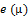is defined as: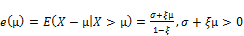(5)

this property is then used as a criterion for the selection of µ.

3.3.2. The Hill Graph

Let x1 > x2 >... > xn be the ordered statistic of random variables iid. The Hill estimator (£) of the tail index using k+1 ordered statistics is defined by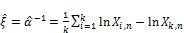(6)

Where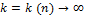is upper order statistics (the number of exceedances), n is the sample size and α=1/ξ is the tail index. The threshold u is selected from this graph for the stable areas of the tail index. However, the choice is not always clear. In fact this method applies well for GDP of close to GDP distributions.

3.4. Parameter Estimation

The maximum likelihood method for estimating parameters for a statistical model was used. In this method the probability density function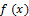can be unknown but the joint density function for the data is assumed to come from a known family of distributions. For an independent and identically distributed sample of size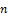the joint density function looks like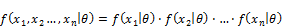(7)

whereare the parameters of the model and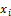are the observed variables. Thus the observed variablesare known whereas the parameters given byare to be estimated. The likelihood function is then given by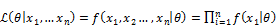(8)

And the often used log-likelihood function is given by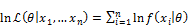(9)

The estimated parameters are then given by the set which maximizes the likelihood function, equation (8) or (9).

4. Results and Discussion

This chapter gives a step by step procedure that was used to estimate extreme value at risk for the prices of US Dollar in Kenyan Shillings using extreme value approach.

Returns were calculate from the prices using the formula:

ri =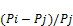(10)

where ri is the return at time i, Pi is the price at time i and j = (i− 1).

The major advantage of using returns is normalization which aids in measuring all variables in a comparable metric. It is thus possible to evaluate analytical relationships between two or more variables even when they originate from unequal valued prices. Most machine learning techniques and multidimensional statistical analysis have this requirement. Further, the log returns are calculated which have both theoretical and algorithmic benefits in usage.

Kenyan Shilling versus US dollar exchange rate returns plot are show in figure 1. Fair stability is observed between 2004 and just before 2007. A great drift is observed during 2007 which is attributed to the chaotic elections held then. Consistent instability is then observed between 2008 and 2010. Arguably, this could have been brought about by the global financial crisis as well as the aftermath of the post-election violence. After 2010, prices are fairly stable, with a mild change evident around 2013 when elections were held. Significant volatility is evident due to the upward and downward movement of exchange rate prices.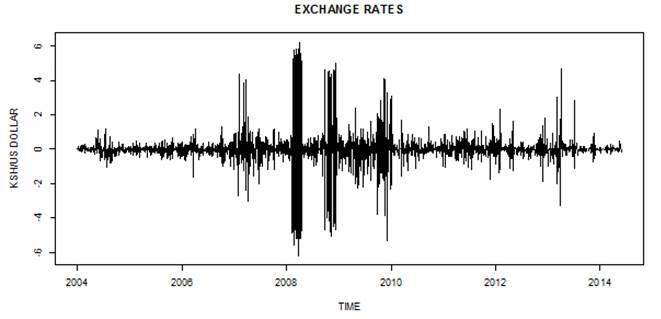Figure 1. Log returns of daily US Dollar Prices.

The basic statistics of raw exchange rate prices are shown in table 1. The Statistics shown above give a mean of 80.40 with a maximum of 106.15 and a minimum of 61.50.the interquartile range is 10.3. A time series plot of prices shows properties such as tread, cyclic and seasonal movements as shown in figure 2.

Table 1. Summary Statistics Exchange Rate Prices.

 Statistic Index Data Minimum 2004-11-01 61.5 1st Quartile 2007-06-01 75.15 Median 2010-01-17 78.88 Mean 2010-01-17 80.40 3rd Quartile 2012-08-26 85.85 Maximum 2015-04-05 106.15

ADF test was carried out to test stationarity of prices as well as those of returns as shown in table 2.

Table 2. ADF Test for Prices and Returns.

 Statistic P-Value Prices -1.9622 0.5943 Returns -14.869 0.01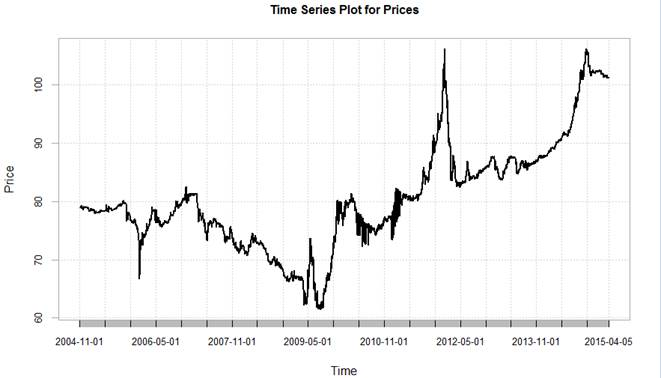Figure 2. Time Series plot prices.

The p-value for prices is greater than the level of confidence and thus we reject the null hypothesis that the series is non-stationary. For the returns we fail reject the null hypothesis at 5 percent level of confidence. Hence returns are stationary.Figure 3. Histogram for Exchange Rates Returns.

4.1. Descriptive Statistics of Returns

Table 3 shows the descriptive statistics of exchange rate returns. The mean return for the series is 0.0000977777 while the standard deviation is 0.008085453. The kurtosis for the data is 24.57076, which is greater than 3 and hence the data is leptokurtic as compared to the normal distribution. This suggests presence of heavy tailed data. The distribution of returns is normal and positively skewed as the skewness is greater than 0. Again, positive skewness implies that the right tail is heavier than the left tail. This is also observable from a histogram of returns as shown in figure 3.

Table 3. Descriptive Statistics Returns.

 Length -0.05911 Mean 9.77777e-05 Maximum 0.06648 Variance 6.53746e-05 Skewness 0.331197 Kurtosis 24.5708

Further, a J-B test was carried out to test for normality of the return series and the statistic was found to be sufficiently large. Hence we reject the null hypothesis that the data is normally distributed. The data is thus heavy tailed.

Correlograms for the returns are drawn as shown in figure 4. Most of the spikes are outside the 95 percent band and thus we reject the null hypothesis that there is no serial correlation in the data. However, there is significance at lag 1. More lags are significant at 5 percent confidence level for the squared returns, thus we conclude that there is serial correlation. This prompts heteroskedastic modeling of the data.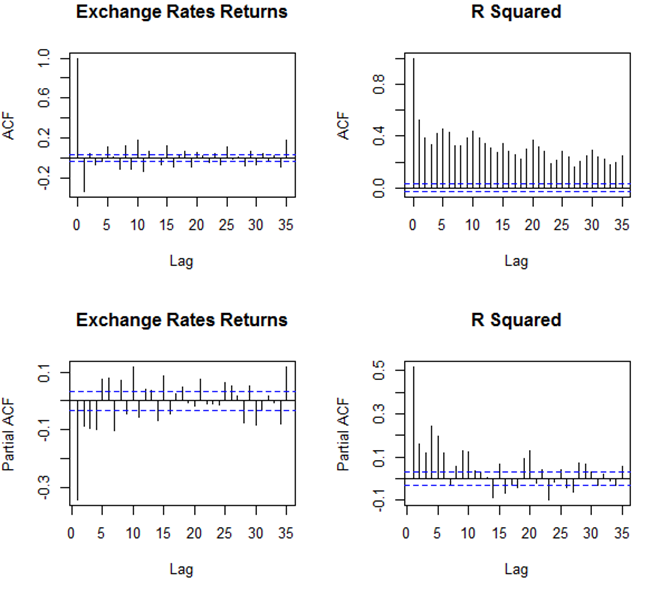Figure 4. Correlograms for Returns.

4.2. Fitting of Heteroskedastic Model

A GARCH model was fitted at different lags and AIC used to select the appropriate model. In addition, the asymmetric GJR-GARCH and E-GARCH models were also fitted estimated to cater for asymmetry in the data. Table 4 represents the estimated parameters as well as AIC for the models. The p-values for the estimated parameters are given in parenthesis. The AIC for the four estimated models differ by a small margin. But a close examination shows the GARCH (1, 1) model to be having significant parameters at 5% level of significance and hence is considered to model the volatility process.

Table 4. Estimated Parameters GARCH model.

 µ α1 α2 β1 β2 AIC 0.000103 0.137848 0.861152 -8.185989 0.000082 0.204297 0.318691 0.476005 -8.187165 (0.90352) (0.45901) (0.83184) (0.66318) 0.000104 0.137889 0.000000 0.861111 -8.184856 0.000093 0.182387 0.061287 0.072025 0.683301 -8.189373 0.000083 0.203646 0.326865 0.468488 0.000000 -8.186028

4.3. Estimated GPD Model

Estimated parameters of the GPD model are show in table 5

The shape parameter estimates indicate that the left tail is heavier than the right tail. Figures 5 and 6 show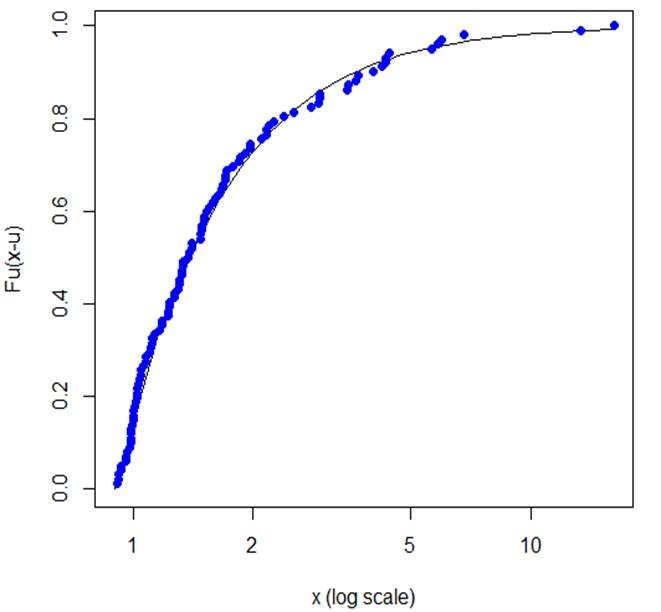Figure 5. Mean Exceedances Fit Left Tail.

the fits of the exceedances to the fitted GPD model. Most of the points lie along the fitted curve and thus the model is generally a good fit to the exceedances of the estimated model. In the Q-Q plots most points lie on the straight line but with some outliers. We can thus conclude that the model is adequate.

Table 5. GPD Model Estimated parameters.

 Threshold (µ) No.of Exceedances £ β Right tail 0.75 488 0.1412458 0.5950302 Left Tail 0.9 477 0.5724507 0.5683319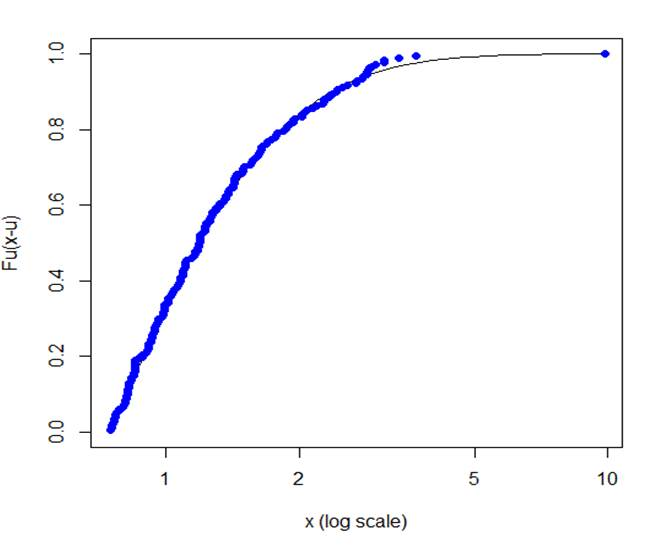Figure 6. Mean Exceedances Fit Right Tail.

4.4. Evaluation of Risk Measures

Table 6 shows the point estimates of risk measures evaluated at relatively high probabilities. The results in the table above indicate that at a level of confidence of 99.0 percent (tail probability of 0.01), the VaR for the right and left tails are 3.553422 and 5.638720 respectively. This implies that with a financial position of 100 Kenyan Shillings, the worst loss that can be experienced is of Shs.3.5534 and Shs. 5.6387 respectively.

Table 6. Estimated Risk measures.

 Probability (p) VaR (p) ES (p) Right Tail 0.9900 3.553422 4.731567 0.9950 4.284900 4.592171 0.9990 6.307591 7.971922 0.9995 7.340929 9.187673 0.9999 10.19826 12.549480 Left Tail 0.9900 5.68720 13.31273 0.9950 8.430245 19.84186 0.9990 21.32235 49.99531 0.9995 31.75524 74.39060 0.9999 79.92231 187.0528

The ES values in the table implies that given VaR is exceeded the expected loss would be of 4.7316 and 13.3127 respectively for the right and left tails. The smaller values in the right tail can be explained from the fact that the left tail of the exceedances was heavier than the right tail. Similarly, with a tail probability of 0.005 the VaR values for the right and left tails are 4.284900 and 8.430245 respectively. Given that these values are exceeded, the expected shortfall values are thus 5.592171 and 19.84186. This values increase with increase in the tail probabilities.

This property is very important in risk evaluation in the finance industry for purposes of hedging.

5. Conclusion and Recommendations

5.1. Conclusion

Implementation of effective risk measures is essential due to the presence of high volatility of exchange rates causing unpredictability. The two stage approach to EVT estimation of the McNeil and Frey (2000) was applied in this research paper. EVT provides a reliable statistical criterion that is useful in modeling risk related to tail distribution of financial data. Use of EVT helps in evaluating the heavy-tail behavior of exchange rates while separating the right tail from the left tail helps in exploration of asymmetric properties. For the choice of threshold, this study considered the mean excess function and the peak over threshold method. The peak over threshold method is favored as it tends to utilize data well. The drawback of this method is a question on the appropriateness of the threshold used.

By assessing the empirical excess distribution functions with associated theoretical distribution, we conclude goodness of fit in modeling tail related data. Extreme tail risk measures are estimated at relatively high levels of confidence such as 99, 99.5, 99.95 and 99.99 percent. EVT-based VaR approach used offers quantitative information for analyzing the extent of potential extreme risk of exchange rates. For an investor this risk measures would important for hedging purposes as well as investment decision making.

5.2. Recommendations

We recommend consideration of EVT in calculation of risk measures due to the high volatility of exchange rates. We also recommend extension of parameter estimation of EVT models under consideration of economic and political factors as they happen to greatly influence volatility of exchange rate prices.

References

1. Bollerslev, T. (1986). Generalized autoregressive conditional heteroskedasticity. Journal of economet- rics, 31 (3):307–327.
2. Castillo, E. and Hadi, S. (1999). "fitting the generalized pareto distribution to data". Journal of the American Statistical Association, 92 (440):1609–2000.
3. Christoffersen, P. and Diebold, F. (2000). How relevant is volatility forecasting for financial risk management? Review of Economics and Statistics 82, pages 1–11.
4. Diebold, F.X., S. T. and Stroughair, T. (1970). Pitfalls and Opportunities in the use of Extreme Value Theory in Risk Management, in Decision Technologies of Computational Finance. Kluwer Academic Publishers, 2 (3-12) edition.
5. Embrechts, P. and Klu¨ppelberg, C. (1997). T. mikosch , modelling extremal events for insurance and finance. Applications of Mathematics-Stochastic Modelling and Applied Probability, 33.
6. Engel, R. (1982)."autoregressive conditional heteroskedasticity with estimation of the variance of u.k. inflation". Econometrica, 50:987–1008.
7. Ng, V. (1993)."measuring and testing the impact of news on volatility". 48:1749–1778.
8. Gencay, R., S. F. (2004). Evt and value at risk relative performance in emerging markets. International Journal of Forecasting, 20:287–303.
9. Engle, R.F., D. L. and Robins, R. (1987)."estimating time-varying risk premia in the term structure".
10. Econometrica, 55:391–408.
11. Engle, R. and HO, L., B. P. C. J. and Theobald, M. (2000)."value-at-risk: Applying the extreme value approach to asian markets in the recent financial turmoil". Pacific Basin Finance Journal, 8:249–275.
12. Jodeu, D. and de Vries, C. (2000). Value-at-Risk and Extreme Returns., volume 60.
13. Kellezi, E. and Gilli, M. (2000). Extreme value theory for tail-related risk measures. FAME, 3.
14. Koedijk, K.G. Schafgans, M. and de Vries, C. (1990). The tail index of exchange rate returns. Journal of International Economics, 29:93–108.
15. L.Glosten, R. J. and D.Runkel (1993). The relationship between the expected value and volatility of nominal excess returns. Journal of finance, 48:1779–1801.
16. Nelson, D. (1991)."conditional heteroskedasticity in asset returns:a new approach". Econometrica, 59:347–370.
17. P. Embrechts, C. K. and Mikosch., T. (1999). Extremal Events for Insurance and Finance. Spring- Verlag, Berlin, 2nd ed. edition.
18. Poon, S.-H. and Granger, C. (2000). Forecasting financial market volatility–a review, proposal pre- sented at the 2001 annual meeting of the american finance association and accepted by the journal of economic literature and the journal of economic survey.
19. Reiss, R.-D., Thomas, M. and Reiss, R. (2007). Statistical analysis of extreme values, volume 2. Springer.
20. Stock, J. and Watson, M. (2007). Introduction to Econometrics. Boston: Addison Wesley, 2nd ed edition.
21. Tsay, R. (2005). Extreme Value, Quantile Estimation and Value at Risk. Hoboken, New Jersey: John Wiley and Sons. Inc, 2nd ed (287-316) edition.
22. Zivot, E. and Wang, J. (2007). Modeling financial time series with S-Plus, volume 191. Springer Science & Business Media.

 Contents 1. 1.1. 1.2. 1.3. 1.4. 2. 3. 3.1. 3.2. 3.3. 3.4. 4. 4.1. 4.2. 4.3. 4.4. 5. 5.1. 5.2.
Article ToolsAbstractPDF(327K)